## 5701 Reputation

8 years, 306 days

## why dsolve missed this singular solution...

Maple 2020

Should dsolve has missed this solution? I used singsol=all option:

```restart;
ode:=y(x)^2+(x^2+x*y(x))*diff(y(x),x) = 0;
dsolve(ode,y(x),singsol=all)
```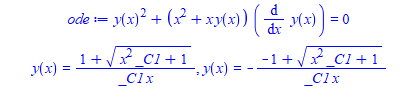But y(x)=0 is singular solution. It can not be obtained from the above general solutions for any constant of integration value.

```odetest(y(x)=0,ode)

0```

if I change the type of ode, Maple now gives y(x)=0 but the general solution is too complicated (which is OK) but the point is that it does now find y(x)=0.

```dsolve(ode,y(x),[dAlembert]);

y(x)=0,  etc.....```

my question is: Why singsol=all did not also give y(x)=0 in this example?  Is there something I am misundertanding here?

Maple 2020.2

## question on using algsubs or an alternat...

Maple 2020

I just had enough with odetest hanging (even when using with timelimit). I wait hours and hours each time (even though I have 30 second timelimit, which Maple ignores) and I do not think Maplesoft is going to fix this in my lifetime.

So I am attempting to make my own very simple and basic odetest.

I give it an ode and explicit solution in for form sol:= y(x)=... and the function uses algsubs(sol,ode) and checks it is gets zero or not (it will also do simplify if needed)

But there is a BIG problem.   Even though algsubs(z=0,z/z);  gives back 1 as expected,  but

```restart;
ode:=diff(y(x), x)/y(x);
sol:=y(x)=0;

algsubs(sol,ode);   #this gives ZERO. It should be 1

```

If we do algsubs on each term one by one

```algsubs(sol,numer(ode));
algsubs(sol,denom(ode));

0
0```

So why did algsubs give zero in the first case, since  the result of the algsubs should be 0/0 which algsubs knows in the limit it is 1?   How did it come up with zero?

Clearly my simple method of replacing odetest with algsubs is not working. I need a more robust way to handle this.

subs does not work. Since subs does not know how substitute y(x)=f(x) into derivatives involved in an ODE.

My question is: Is there a way to teach algsubs to give 1 for the above example? or better function to use?

I tried applyrule instead of algsubs, but that does not work.

```applyrule(sol, ode)
```

Error, (in rec) numeric exception: division by zero

Is there a better method to use? I am trying to do simple version of odetest that does not hang. Even if not perfect. Will only use it for explicit solutions, not implicit since implicit is much harder.

Here is a more full example

```ode:=diff(y(x),x)^2+2*x*diff(y(x),x)/y(x)-1 = 0;
sol:=y(x)=0;
odetest(sol,ode)```

0

But when I use my simple method

```ode:=diff(y(x),x)^2+2*x*diff(y(x),x)/y(x)-1 = 0;
sol:=y(x)=0;
algsubs(sol,ode);```

-1 = 0

The reason it failed, because algsubs replaced the second term by 0 instead of 1. The second term in the ode is diff(y(x),x)/y(x)

Which is 0/0 but this is 1 in the limit. But algsubs used 0 instead for some reason.

So I need a little bit smarter way to replace my solution into the ode than just using algsubs. May need to use some of the tricks I've seen used here before using freez/thaw/frontend, etc.. which I still do not understand.

Any recommendation?  This is meant to work for any single ode and any explicit solution of the form y(x)=....

This algsubs method works actually pretty well on many ode's. I've tested it on 2,000 ode's. It just fails so far on subtle ones like the above. Here is just random example where it works

```restart;
ode:=diff(y(x),x)^2 = (-x+1)/x;
sol:=y(x)=_C1+arcsin(2*x-1)/2+sqrt(x-x^2);

evalb(simplify(algsubs(sol,ode)))
```

true

So I just need a way to handle the cases where it gives 0/0 I think. I have 20 ODE's which now fail out of about 2,000 using this basic method compared to using odetest.

Thank you

## why simplify works on A-B giving zero, b...

Maple 2020

I was trying to simplify long expression of the form    f(x)=g(x), where f(x) should simplify to be the same as g(x) and get 0=0. But Maple simplify just return the input back.

Then I wrote simplify(f(x)-g(x)) and now Maple returned 0 back.

What is the difference between simplify (f(x)=g(x)) and simplify(f(x)-g(x))? And why the second worked but not the first?

```restart;
f:=(9*(x^(-2/3*a))^2*exp(6/a*(x^(-2/3*a))^(1/2))^2*_C0^2-6*(x^(-2/3*a))^(3/2)*exp(
6/a*(x^(-2/3*a))^(1/2))^2*_C0^2*a+x^(-2/3*a)*exp(6/a*(x^(-2/3*a))^(1/2))^2*_C0^
2*a^2+18*(x^(-2/3*a))^2*exp(6/a*(x^(-2/3*a))^(1/2))*_C0-2*x^(-2/3*a)*exp(6/a*(x
^(-2/3*a))^(1/2))*_C0*a^2+6*(x^(-2/3*a))^(3/2)*a+x^(-2/3*a)*a^2+9*(x^(-2/3*a))^
2)/(3*_C0*exp(6/a*(x^(-2/3*a))^(1/2))*(x^(-2/3*a))^(1/2)-exp(6/a*(x^(-2/3*a))^(
1/2))*_C0*a+3*(x^(-2/3*a))^(1/2)+a)^2:

g:=x^(-2/3*a):
```

And now

`simplify(f=g);`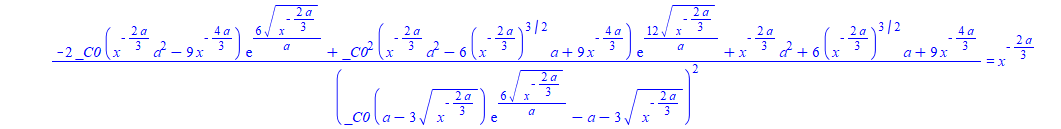But

`simplify(f-g);`

0

And

`simplify(f-g=0);`

0 = 0

Why Maple behaves like this? I did not know it makes difference if one writes f=g vs. f-g in terms of simplification.

I copied the code to Mathematica to see how it behaves, and Mathematica Simplify worked on both f=g and also on f-g as one would have expected: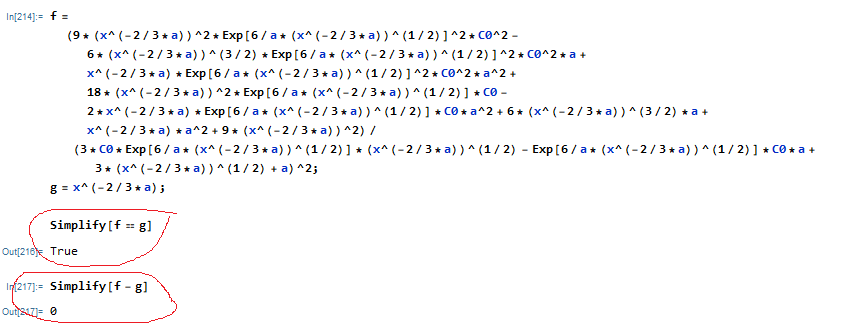Maple 2020.2 on windows

## what is the difference between doing res...

Maple 2020

For the last hr, I've been trying to find out why Maple solve was hanging inside one module in my .mla when called.

I would try the same code in new worksheet, no hang. I had timelimit on it. Same exact code as in the module.

I go back to the other worksheet, do restart, call the module (after loading the library by setting libname) which calls solve(), and it hangs. Each time.

I tried may be 10 times. No difference between the code in the mla and the other worksheet where I had copy of the solve command. All other settings are the same.

ONLY after I closed Maple, and restarted Maple, and opened the worksheet where the call used to hang, and called the module again, now it did not hang and solve completed.

This is not the first time I've seen such a strange thing. Sometimes Maple will hang for hrs (I start  a test, go to sleep,. wake up hrs later, and see maple hanged for hrs from the time stamp).

Closes Maple, start the test from where it was hanging, and now no hang. It runs OK from then on.

This tells me that doing just restart from the worksheet do not clean everything. (I use the command restart, not by pressing the restart button in the menu, not sure if these are different, they should be the same  I would think).

I've seen this problem before. sometimes the test just hangs. No matter how many time I do restart from the worksheet, it just hangs. When I restart Maple, it works from same place it was hanging.

My question is, what could possibly make Maple not hang when starting fresh. vs. using the restart command?  I am using the same worksheet, and I have "create new engine for each document" selected in options.

The hang used to happen in solve, in here

```#this is inside a function inside a module inside .mla library
#

eq:=x = 1/2/(p^2+2)^(1/8)*2^(1/2)*((1+(-4*p^2+1)^(1/2))/(1/(4*p^2-1)*p^2)^(1/2)/(-4
*p^2+1)^(1/2))^(3/4)/(((-4*p^2+1)^(1/2)+3)/(p^2+2)^(1/2))^(1/4)*_C1*p^(1/4);

try
sol_p:=timelimit(20,[:-solve(x=rhs(eq),p)]);
catch:
return [];
end try;
#hangs here. Never completes. Only when starting Maple the hang is gone.

#trace below
......
Main: polynomial system split into 1 parts under preprocessing
Main: using RegularChains based methods
SolverVariableOrder: using the variable order  _S000008 > _S000011 > _S000009 > _S000006 > _S000005
TriangularDecomposition: using deterministic algorithm for decomposition
<<<< HANGS HERE EACH TIME >>>>```

Again, only when I restarted Maple, did the hang go away. it is one equation solving for one variable.

So now, I get into the habit of closing all of Maple and starting it again when I get a hang. I do not trust restart command any more to clear everything.

Any idea why this happens? Memory not cleared somewhere? Cache problem? Does the frontend itself stores something that could cause this? does restart use new mserver.exe each time or same mserver.exe process as last time?

if restart does not actually terminate the mserver.exe that the worksheet was talking to, but only sends request to mserver.exe to clear its own memory, this could explain things.

It is possible that mserver.exe which is attached to that worksheet does not fully clear all its own memory and something remain there which affects why it hangs in same place each time, even though restart was issued each time, and only when restarting Maple itself will clear this and the hangs goes away.

## odetest does not handle some solutions f...

Maple 2020

When asking dsolve to use specific ode type to solve an ode, in particular, dAlembert type, which typically generate complicated solutions, sometimes dsolve solution shows up using parameter T.

But odetest gets confused by this expression it seems. I am not able to figure if I am doing something wrong in using odetest, or may be odetest does not know how to handle such form of a solution.

Here is an example. This ode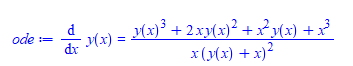is of these types:

```restart;
ode:=diff(y(x), x) = (y(x)^3 + 2*x*y(x)^2 + x^2*y(x) + x^3)/(x*(y(x) + x)^2);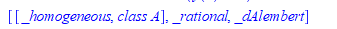By default, dsolve was smart to use homogeneous type to solve the ODE, as this gives the simplest solution.

One can force dsolve to use the other types. When using dAlembert, odetest gives an error trying to verify any one of the solutions returned from dsolve due to the way the solution is returned. Here is the result

```restart;
ode:=diff(y(x), x) = (y(x)^3 + 2*x*y(x)^2 + x^2*y(x) + x^3)/(x*(y(x) + x)^2);
sol:=[dsolve(ode,y(x),[homogeneous])];
```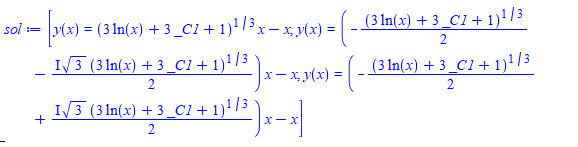No problem here for odetest. it can verify any of the above 3 solutions with no error generated.

```odetest(sol,ode)

0```

Lets compare using dAlembert type

```restart;
ode:=diff(y(x), x) = (y(x)^3 + 2*x*y(x)^2 + x^2*y(x) + x^3)/(x*(y(x) + x)^2);
sol:=[dsolve(ode,y(x),[dAlembert])]: #solution too complicated to show here
odetest(sol,ode)
```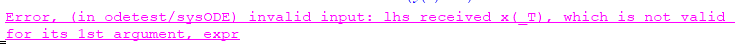It looks like odetest does not know how to handle the form of the solution as returned by dsolve for this case. The problem is that each solution is actually made up of two parts, not just y(x) as normally is the case. One part defines something called X(T_) and the next part which is the solution y(x) uses this X(T) in it.

Did I do something wrong, or is there a way around this, or is this by design?

Maple 2020.2

﻿